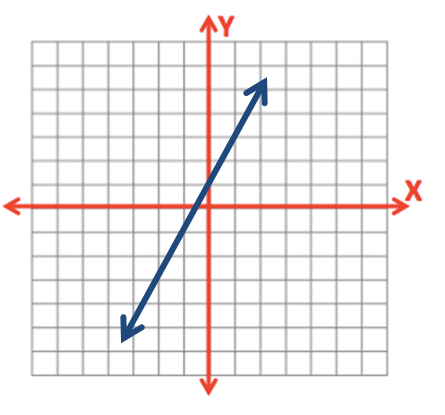# 0.1 - Review of Algebra

0.1 - Review of Algebra

Knowledge of the following mathematical operations is required for STAT 200:

• Addition
• Subtraction
• Division
• Multiplication
• Radicals (square roots)
• Exponents
• Summations  $$\left( \sum \right)$$
• Basic linear equations (i.e., $$y=mx+b$$)

The ability to perform these operations in the appropriate order is necessary. The following sections will review order of operations, summations, and basic linear equations.

In the past, students without knowledge of these topics have struggled to be successful in STAT 200. If you have any questions about these mathematical operations after reviewing these materials, please contact your instructor as soon as possible. We want our students to be successful!

# 0.1.1 - Order of Operations

0.1.1 - Order of Operations

The acronym PEMDAS, or the mnemonic "please excuse my dear aunt Sally," are sometimes used to help students remember the basic order of operations, where P = parentheses, E = exponents (and square roots), M = multiplication, D = division, A = addition, and S = subtraction.

When performing a series of mathematical operations, begin inside the parentheses. Next, calculate any exponents or square roots. Then, multiplication and division. And finally, addition and subtraction. For a more in-depth review, we recommend the Khan Academy's Order of Operations lesson.In this course, we will be using fractions often. When working with fractions, you can imagine that the operations in the numerator are within parentheses and the operations in the denominator are in parentheses. Below are a few examples of mathematical operations that will be applied in this course. We'll learn about the applications of these operations later in the course, here, we're focusing only on the mathematical operations.

## Example: Confidence Interval for a Mean

A confidence interval for a mean can be computed using the equation $$\bar x \pm SE (t^*)$$. Let's construct a confidence interval given $$\bar x = 5.770$$, $$SE = 0.335$$, and $$t^* = 2.080$$.

First, we'll plug in the given values.

$$5.770 \pm 0.355(2.080)$$

There are no operations within parentheses and no exponents or square roots, so our next step will be to multiply.

$$5.770 \pm 0.697$$

The symbol ± tells use to both subtract and add.

$$5.770 - 0.697 = 5.073$$

$$5.770 + 0.697 = 6.467$$

The confidence interval is (5.073, 6.467).

## Example: Test Statistic for a Proportion

A test statistic for a one sample proportion hypothesis test can be computed using the equation $$z = \frac {\hat p - p_0}{\sqrt{\frac{p_0 (1-p_0)}{n}}}$$. Let's work through this formula using the following values: $$\hat p = 0.87$$, $$p_0 = 0.8$$, and $$n=100$$.

First, we'll plug in the given values.

$$z = \frac {0.87 - 0.8}{\sqrt{\frac{0.8 (1- 0.8)}{100}}}$$

In the first few steps we'll work in the numerator and denominator separately. Let's start in the numerator, which contains only subtraction.

$$z = \frac {0.07}{\sqrt{\frac{0.8 (1- 0.8)}{100}}}$$

Next, let's focus on the denominator. The operation within the parentheses should be conducted first.

$$z = \frac {0.07}{\sqrt{\frac{0.8 (0.2)}{100}}}$$

Within the denominator, we can work within the top of the fraction first,

$$z = \frac {0.07}{\sqrt{\frac{0.16}{100}}}$$

Solve for fraction under the square root in the denominator.

$$z = \frac {0.07}{\sqrt{0.0016}}$$

Next, take the square root in the denominator.

$$z = \frac {0.07}{0.04}$$

And finally, divide the numerator by the denominator.

$$z = 1.75$$

# 0.1.2 - Summations

0.1.2 - Summations

$$\Sigma$$

This is the Greek capital letter "sigma." In math, this symbol is also known as a summation. This tells us that we should add a series of numbers (i.e., take the sum).

## Example: Candy

Four children are counting how many pieces of candy they each have. They want to know how many pieces of candy they have all together.

Child Pieces of Candy
Jenny 9
Carrie 8
Eugenia 10
Kevin 8

If we wanted to know how many total pieces of candy the group of children had, we could add these four numbers. The notation for this is $$\sum x_{i}$$.

$$\sum x_{i}=9+8+10+8=35$$

Combined, the four children have a total of 35 pieces of candy.

# 0.1.3 - Basic Linear Equations

0.1.3 - Basic Linear Equations

You may recall from an algebra class that the formula for a straight line is $$y=mx+b$$ where $$m$$ is the slope and $$b$$ is the y-intercept. The x-axis is the horizontal axis and the y-axis is the vertical axis.The y-intercept is where the line crosses the y-axis.  The slope is a measure of change in y over change in x, sometimes written as $$\frac{\Delta y}{\Delta x}$$ where $$\Delta$$ ("delta") means change.

## Example

$$y=2x+1$$

Here, the y-intercept is 1 and the slope is 2.  This line will cross the y-axis at the point (0, 1). From there, for every one increase in $$x$$, $$y$$ will increase by 2. In other words, for every one unit we move to the right, we will move up 2.In statistics, the y-intercept is typically written before the slope. The equation for the line above would be written as $$y=1+2x$$.

Different notation may also be used:

$$\widehat y = a + bx$$, where $$\widehat y$$ is the predicted value of y, $$a$$ is the y-intercept and $$b$$ is the slope.

$$\widehat y = b_0 + b_1 x$$, where $$\widehat y$$ is the predicted value of y, $$b_0$$ is the y-intercept, and $$b_1$$ is the slope.

  Link ↥ Has Tooltip/Popover Toggleable Visibility